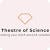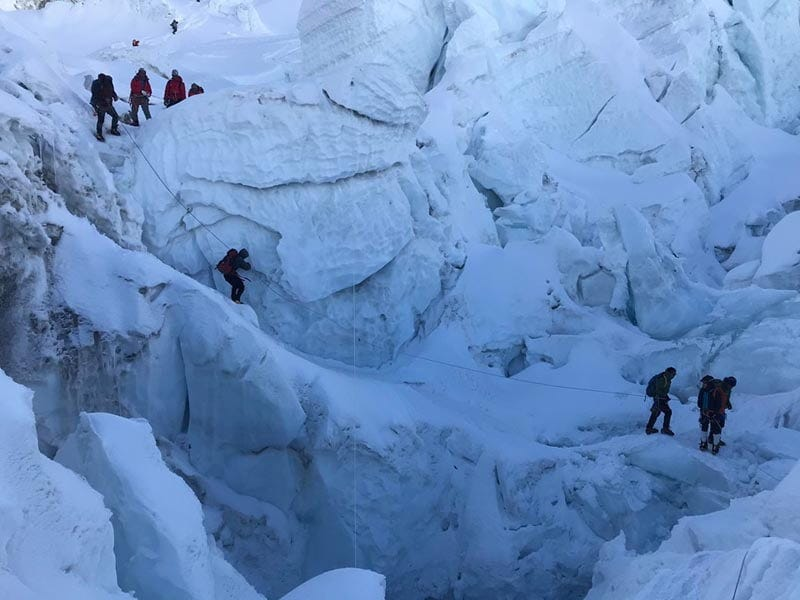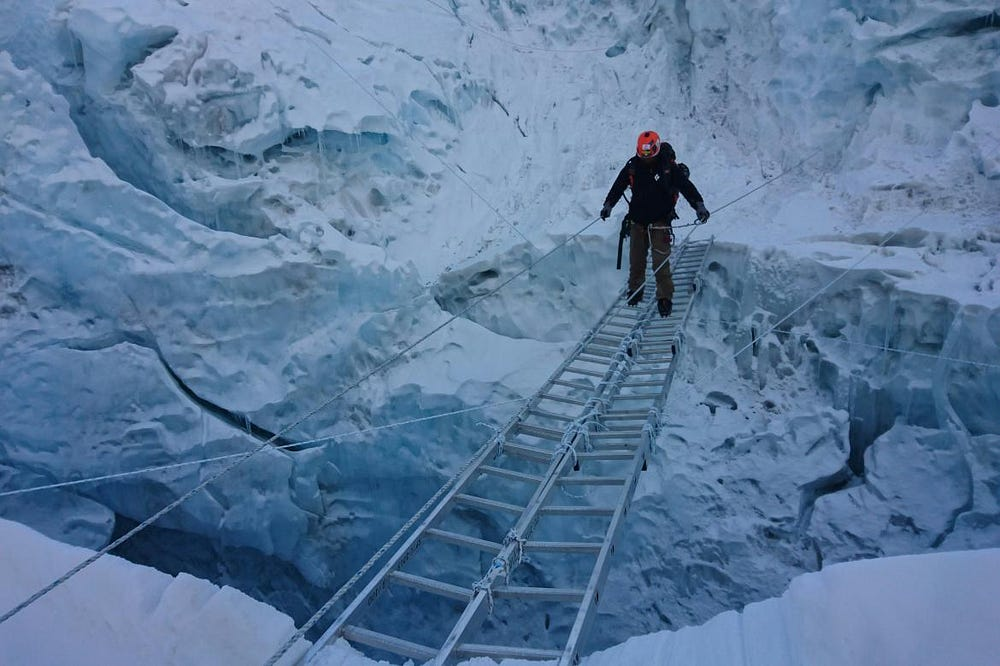Playing with Gradient Descent Intuition

Source: Deep Learning on Medium

Before going to the topic let’s understand some basics and common sense questions ?

okay so imagine you are on some mountain (maybe mount Everest or K 2)

Now when you were thinking of coming down then weather was not according to your mood and there were clouds all over and you can’t see anything. Now in that situation what would you do ?

Will you sit there crying and waiting for help arrival ? Okay that maybe one option but think about when there can’t be any help because choppers can’t reach at that top and you have to come down at least for some kilometres.

Now what you do in that situation ?
If your guess is to try and look around locally good solution then it’s the right path. Maybe the locally optimal solution will take us to globally optimal solution. Let’s see if that happens in a moment. So , you start descending down where you see down slope and finally reach the point where the help arrived.

One more example is in software engineering where we had to rapidly prototype the product and then make it and release in the public. Then we get the feedback and then we correct the bugs and then keep doing it until the product is bug free or at user convenience.

How did that worked.
Let’s understand that in mathematical way first.

In above examples we can observe that we want to find some physical quantity to be minimum (height in first example and bugs in second example).The quantity is continuous in nature (meaning it changes with time).That means that quantity is functional ( function of anything).

Now we have build up ready for coming part.

According to wikipedia : “Gradient descent is a first-order iterative optimization algorithm for finding the minimum of a function. To find a local minimum of a function using gradient descent, one takes steps proportional to the negativeof the gradient (or approximate gradient) of the function at the current point. If, instead, one takes steps proportional to the positive of the gradient, one approaches a local maximum of that function; the procedure is then known as gradient ascent. Gradient descent is also known as steepest descent.

Let’s understand that when we try to find the minima of a function then what do we do we differentiate the function by taking derivative of that function and then try to find critical points (where function changes it’s value) and then check the condition for double derivatives (negative for finding maxima and positive for finding minima) and we get that point.

Now according to gradient descent how do we reach that point ?

consider this function :

whose gradient (partial derivative w.r.t to all variables -here only x ) is :

now , let’s start with some random weight (x₀) = 5.

then the question is in which direction to move and why in that direction ?[keep going , you are good to go]

Since we know that gradient of a function for a vector space gives always the direction of greatest rate of increase and so we need to move in the negative of that direction to minimize the value of the function.

How much to move ? In technical terms , we can say that to be our learning rate. (say for here , 0.001).

Let’s go —

find out the next point –

x₁ = 5+ 0.001* (-)(4*6³- 9*6²) = 4.725

after 468 iterations , we get minimum at 2.250048101595436 (according to the precision we set)

Now , we saw gradient descent mathematical intuition.hey , you reached down !

Hurray ! you reached to bottom of mount everest ! and now you can be seen and help can be reached.

but wait while going down , you seem to stuck in a valley where you can’t really see the locally down slope direction and so now what will you do ?[ aw ! you are trapped now]

but don’t worry !

for now you are rescued !

(it’s that moment when you can say “i beleive in maths”)